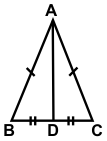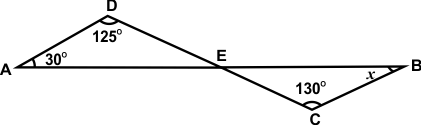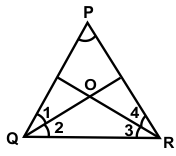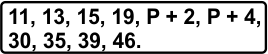# SOF - IMO Level 2 Sample Papers for Class 9

Class 9 sample paper & practice questions for the level 2 of International Mathematics Olympiad (IMO) are given below. Syllabus for level 2 is also mentioned for these exams. You can refer these sample paper & quiz for preparing for the exam.#### Resources:

##### Sample Questions from Olympiad Success:
 Q.1 Q.2 Q.3 Q.4 Q.5 Q.6 Q.7 Q.8 Q.9 Q.10
 Q.1 In the given figure, if AB = AC & BD = DC, find the measure of ∠ADC.a) 60° b) 120° c) 90° d) 110°
 Q.2 If X, Y, Z are three points on a line and Y lies between X and Z, which of the following is true? a) 1/2(XY + YZ) = XZ. b) XY + XZ = YZ. c) XZ + YZ = XY. d) XY + YZ = XZ.
 Q.3 What is the rationalizing factor of 5√(a2b3c4? a) 5√a3b2c b) 4√a3b2c c) 3√a3b2c d) √a3b2c
 Q.4 The first term in a sequence is 1 and the second term is 5. From the third term on each term is the average (arithmetic mean) of all preceding terms. What is the 25th term in the sequence? a) 3 b) 5 c) 2.5 d) None of these
 Q.5 If lines AB and CD intersect as shown below, find the value of angle x.a) 85° b) 25° c) 38° d) 54°
 Q.6 In ∆ PQR, the angle bisectors of ∠PQR & ∠PRQ meet at O.If ∠QPR = 80°, find the measure of ∠QOR.a) 80° b) 130° c) 100° d) 90°
 Q.7 The numbers 1 to 15 are written on 15 pieces of paper and dropped into a box. Three of them are drawn at random. What is the probability that the three pieces of paper picked have numbers that are in arithmetic progression? a) 1.528 b) 0.158 c) 0.116 d) 0.107
 Q.8 The median of given observations arranged in ascending order is 25.Find p.a) 22 b) 24 c) 21 d) 26
 Q.9 Find the coordinates of points which lies on x-axis at a distance of 4 units from origin in the positive direction of x-axis. a) (0, 4) b) (-4, 0) c) (0, -4) d) (4, 0)
 Q.10 If (3, 4) is a solution of the equation 5x - 2y = k, find the value of k. a) 7 b) 6 c) 5 d) 4

 Q.1 )c Q.2 )d Q.3 )a Q.4 )a Q.5 )b Q.6 )b Q.7 )d Q.8 )a Q.9 )d Q.10 )a

Q.1 : c | Q.2 : d | Q.3 : a | Q.4 : a | Q.5 : b | Q.6 : b | Q.7 : d | Q.8 : a | Q.9 : d | Q.10 : a